# A Clear Picture of Basic Arithmetic with Wolfram|Alpha’s Step-by-step

January 27, 2014 —Comments Off

Wolfram|Alpha has been adding more step-by-step functionality to accommodate the needs of students at various levels of education. Now with Wolfram Problem Generator and Step-by-step solutions, students essentially have their own private tutor to help them better understand their homework and advance their knowledge.

The new steps for simple arithmetic are geared toward a younger crowd and use friendly tools like blocks and number lines to help kids better relate to their math problems. So if parents are struggling with how to explain elementary concepts of addition, subtraction, multiplication, or division, they can now turn to Wolfram|Alpha for a kid-friendly, yet detailed explanation.

Let’s start with addition. You and I both know how to add three and four, but the process has become so axiomatic, it’s hard to explain “how” other than to say “just do it.” Here is an example of a Step-by-step solution for 3 + 4.Each step starts out with an engaging hint to help nudge the child in the correct direction. Then follow the actual directions, often involving drawings of blocks. Not every kid may like math, but everybody likes to draw!

Let’s move onto subtraction. So you’re thinking: “OK, it’s not such a bad idea to think of numbers as blocks. But won’t it be confusing for subtraction when blocks just start to disappear?” Fear not, we thought it would be confusing too. Here is an example with 13 – 5.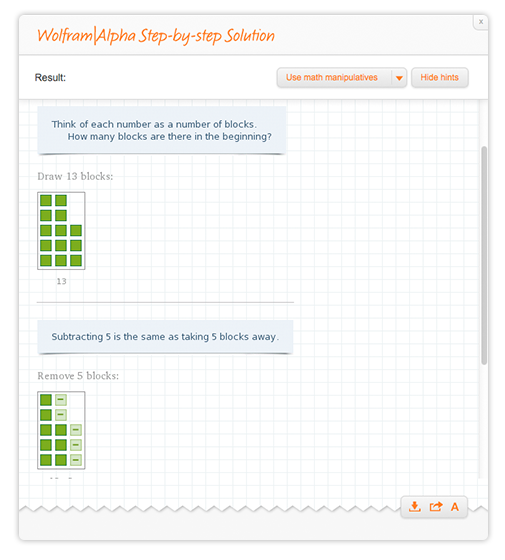As you can see, we use blocks with suggestive minus signs to represent the blocks we have taken away as a result of subtraction. Now the student simply needs to count the number of standard green blocks to see that 13 minus 5 equals 8.

Counting blocks for addition and subtraction seems like a natural tool. But what about for multiplication and division?

We give two different elementary steps to help students understand simple multiplication problems. Let’s look at the example 3 x 4. First we use the usual blocks.Now the kids can resort back to block-counting to find the correct answer.

By the time children are learning how to multiply two numbers, they have had time to practice addition. So the second method helps convert a new and perhaps intimidating multiplication problem into a more familiar addition problem.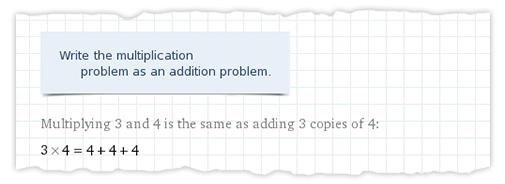The two different methods ensure that different students with different learning techniques can understand the steps. They also help the same student understand that a math problem can be approached multiple ways.

There are steps using blocks to help students understand division as well. For example, the steps for 14 / 3 start out by explaining the procedure of division.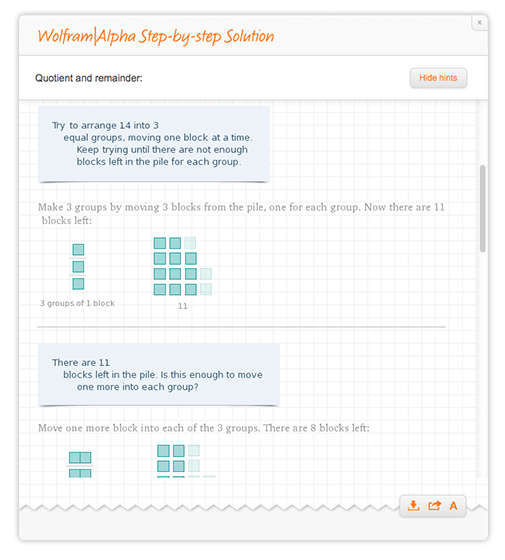Using the method of grouping blocks, kids can physically see the process of division and hopefully be less intimidated by the new operation they have learned.

Counting green blocks can become dull for older kids. There is an addition and subtraction method using the number line for such students. These steps are helpful for simple addition and subtraction as well as slightly more complicated operations such as -8 + 14 or 5 – 23.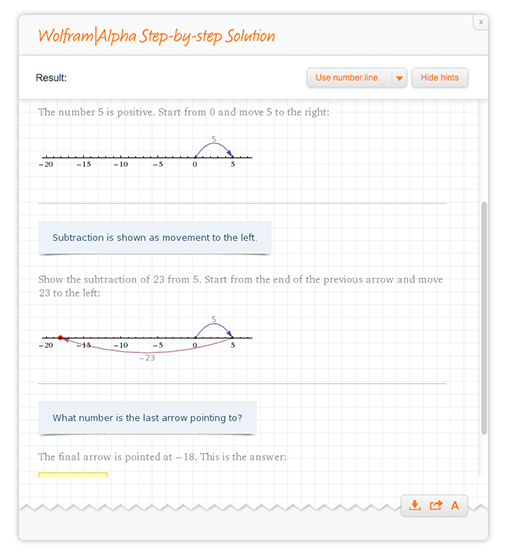Lastly, we now have steps for fraction addition with common denominators, such as 5/7 + 13/ 7, using pie charts.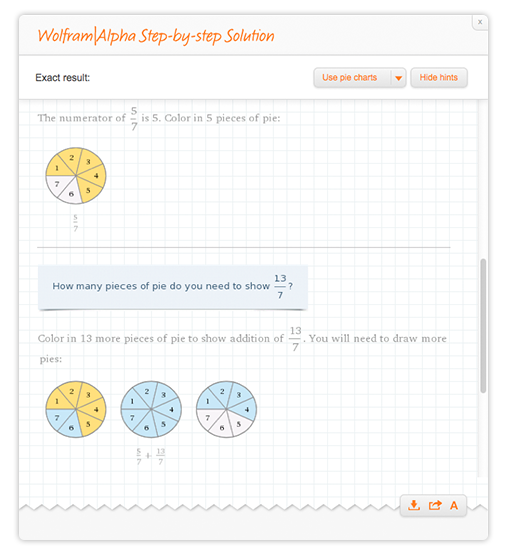The pies help teach the actual mathematical concept behind this strange new way of stacking numbers on top of each other. Plus, it’s always fun being able to draw math!

The new steps and Wolfram Problem Generator together provide parents of younger students the extra resources to help their kids get ahead in math. Now math can be a fun drawing project with your child instead of a chore for both of you!

Excellent article! So easy to understand even a child could get it.

Posted by Ben R January 27, 2014 at 12:49 pm

Oh wow! I never looked at division that way. This is great!

Posted by Jasper January 28, 2014 at 12:26 pm

i am not clear how to get problem generator to generate negative numbers when doing arithmetic.

Posted by joe robinson May 4, 2014 at 12:11 am

Hi Joe,

For now, we do not have negative numbers arithmetic in the problem generator. However, if you’re working on problems and need help, we do have Step-by-step for it!

Posted by Stephanie Oh May 7, 2014 at 9:14 am

excelente me parece muy util, como base de las matematicas quien aprende ha realizar operaciones con fracciones se le faxcilitara todos los siguientes cursos supereiores….

Posted by martha November 12, 2015 at 11:43 pm